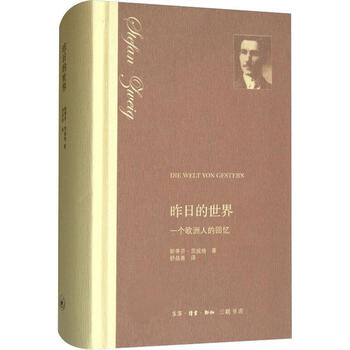# 《昨日的世界 一个欧洲人的回忆》• 店铺： 文轩网旗舰店
• 出版社：生活.读书.新知三联书店
• ISBN：9787108062024
• 商品编码：31949037101
• 品牌：文轩
• 出版时间：2018-06-01

\r\r\r\r\r\r\r\r\r作者:(奥)斯蒂芬・茨威格(Stefen Zweig) 著 舒昌善 译\r\r\r\r定价:68\r\r\r\r出版社:生活.读书.新知三联书店\r\r\r\r出版日期:2018年06月01日\r\r\r\r页数:607\r\r\r\r装帧:精装\r\r\r\rISBN:9787108062024\r\r\r\r\r\r\r\r\r\r\r\r\r●序言
\r章太平世界
\r第二章上个世纪的学校
\r第三章情窦初开
\r第四章大学生活
\r第五章巴黎，永葆青春的城市
\r第六章我的曲折道路
\r第七章走出欧洲
\r第八章欧洲的光辉和阴霾
\r第九章次世界大战爆发时
\r第十章为思想上的团结而奋斗
\r第十一章在欧洲的心脏
\r第十二章回到祖国奥地利
\r第十三章重又走向世界
\r第十四章夕阳西下
\r第十五章希特勒的崛起
\r第十六章和平气息奄奄
\r译者后记\r\r\r\r\r\r\r\r

\r内容简介\r

\r\r\r斯蒂芬・茨威格著的《昨日的世界(一个欧洲人的回忆)(精)》是一部带有自传性质的作品。这本书所展现的是一幅时代的画卷：“一战”前作为文化艺术之都的维也纳的黄金时代；作者与欧洲知识分子、作家、音乐家、艺术家的交往；“一战”时交战各国知识分子狂热的民族主义和爱国主义情绪；战后德奥经济崩溃时的惨象以及纳粹希特勒的崛起及第二次世界大战……作者“出于绝望”，以感人至深的笔触写下的“我一生的历史”，以此纪念一段美好的岁月，并尽一个在文明倒退时代中的“手无寸铁、无能为力的见证人”的义务。\r\r\r\r\r\r\r\r\r\r\r\r\r\r\r\r\r\r\r\r\r